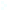Election CitationResearch Interests Name Ribet, Kenneth A. Location University of California, Berkeley Primary Field Mathematics Ribet is a number theorist who did deep work on l-adic representations of Galois groups and modular forms. In addition, Ribet revolutionized the classical subject of cyclotomic fields by introducing a technique of great originality. Ribet is best known for his crucial role in Wiles' proof of Fermat's Last Theorem. I work in arithmetical algebraic geometry (or arithmetic), a field of number theory that uses tools from algebraic geometry to study diophantine problems. Such problems arise when one seeks to identify all solutions to an equation (or family of equations) that are whole numbers, fractions, or other quantities in number theory. For example, whole number solutions to the diophantine equation x^2 + z^2 = 2y^2 correspond to triples of perfect squares, like (1, 25, 49), in which the middle entry is the average of the first and third entries. Although the field of arithmetic uses many technical tools of recent vintage, its study is motivated by some of the oldest problems in mathematics. In the last decade the most notable success of arithmetic was the proof of Fermat's Last Theorem, a simple statement about perfect powers whose demonstration had eluded mathematicians for over 350 years.